# Unipotent element

(diff) ← Older revision | Latest revision (diff) | Newer revision → (diff)
Jump to: navigation, search

An elementof a linear algebraic groupwhich coincides with the unipotent part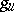of its Jordan decomposition in. Ifis realized as a closed subgroup of the groupof automorphisms of a finite-dimensional vector spaceover an algebraically closed ground field, then a unipotent elementis precisely an elementsatisfying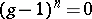,, or, equivalently, is such that its matrix relative to a certain basis ofis upper triangular, with 1's on the main diagonal. The set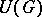of all unipotent elements inis closed in the Zariski topology. If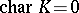, then every unipotent elementhas infinite order. In that case the smallest algebraic subgroup ofcontainingis a one-dimensional unipotent group. If, however,, thenwill be unipotent precisely when it has finite orderfor some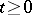. A connected group contains no unipotent element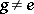if and only if it is an algebraic torus.

A criterion for anisotropy may be given in terms of unipotent elements (cf. Anisotropic group).

Unipotent elements play an important role in the theory of discrete subgroups (cf. Discrete subgroup) of algebraic groups and Lie groups. The presence of unipotent elements in a discrete groupof motions of a symmetric space, having a non-compact fundamental domain of finite volume, is an important tool for studying the structure of such groups and their fundamental domains, cf. ; the existence of unipotent elements in suchwas proved in .

The varietyis invariant under inner automorphisms of the group. Letbe connected and semi-simple. Then the number of conjugacy classes of unipotent elements is finite and for every simplethere is a complete description of them (as well as a description of the centralizer of a unipotent element), cf. . In the classical groups such a description is obtained by means of the Jordan form of matrices, . E.g. for the group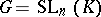there exists a bijection between the conjugacy classes of unipotent elements and the partitions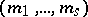ofinto positive integers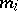,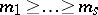. Ifand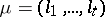are two partitions of, then the class corresponding tocontains in its closure the class corresponding toprecisely if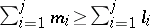for all. The dimension of the class corresponding to the partition(as an algebraic variety) is equal to.

The set of all simple points of the algebraic varietyforms one conjugacy class of unipotent elements, the regular unipotent elements. Ifis simple, then the variety of singular points in the varietyalso contains a Zariski-open conjugacy class of unipotent elements — the subregular unipotent elements. For a study of the singular points of the varietysee also .